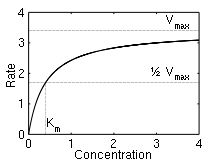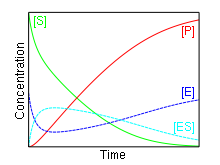# Michaelis–Menten kinetics

Michaelis–Menten kineticsAn example curve with parameters Vmax=3.4 and Km=0.4.

In biochemistry, Michaelis–Menten kinetics is one of the simplest and best-known models of enzyme kinetics. It is named after German biochemist Leonor Michaelis and Canadian physician Maud Menten. The model takes the form of an equation describing the rate of enzymatic reactions, by relating reaction rate v to [S], the concentration of a substrate S. Its formula is given by$v = \frac{ V_\max {[S]}}{K_m + [S]}.$

Here, Vmax  represents the maximum rate achieved by the system, at maximum (saturating) substrate concentrations. The Michaelis constant Km is the substrate concentration at which the reaction rate is half of Vmax . Biochemical reactions involving a single substrate are often assumed to follow Michaelis–Menten kinetics, without regard to the model's underlying assumptions.

## ModelChange in concentrations over time for enzyme E, substrate S, complex ES and product P

In 1903, French physical chemist Victor Henri found that enzyme reactions were initiated by a bond between the enzyme and the substrate. His work was taken up by German biochemist Leonor Michaelis and Canadian physician Maud Menten who investigated the kinetics of an enzymatic reaction mechanism, invertase, that catalyzes the hydrolysis of sucrose into glucose and fructose. In 1913, they proposed a mathematical model of the reaction. It involves an enzyme E binding to a substrate S to form a complex ES, which in turn is converted into a product P and the enzyme. This may be represented schematically as$E + S \, \overset{k_f}\underset{k_r} \rightleftharpoons \, ES \, \overset{k_{cat}} {\longrightarrow} \, E + P$

where kf, kr and kcat denote the rate constants, and the double arrows between S and ES represent the fact that enzyme-substrate binding is a reversible process.

Under certain assumptions – such as the enzyme concentration being much less than the substrate concentration – the rate of product formation is given by$v = V_\max \frac{[S]}{K_m + [S]} = k_\text{cat} [E]_0 \frac{[S]}{K_m + [S]}.$

The reaction rate increases with increasing substrate concentration [S], asymptotically approaching its maximum rate Vmax , attained when all enzyme is bound to substrate. It also follows that Vmax  = kcat[E]0, where [E]0 is the enzyme concentration. kcat, the turnover number, is maximum number of substrate molecules converted to product per enzyme molecule per second.

The Michaelis constant Km is the substrate concentration at which the reaction rate is at half-maximum, and is a measure of the substrate's affinity for the enzyme. A small Km indicates high affinity, meaning that the rate will approach Vmax  more quickly.

The model is used in a variety of biochemical situations other than enzyme-substrate interaction, including antigen-antibody binding, DNA-DNA hybridization and protein-protein interaction. It can be used to characterise a generic biochemical reaction, in the same way that the Langmuir equation can be used to model generic adsorption of biomolecular species.

## Applications

Parameter values vary wildly between enzymes:

Enzyme Km (M) kcat (1/s) kcat / Km (1/M.s)
Chymotrypsin 1.5 × 10-2 0.14 9.3
Pepsin 3.0 × 10-4 0.50 1.7 × 103
Tyrosyl-tRNA synthetase 9.0 × 10-4 7.6 8.4 × 103
Ribonuclease 7.9 × 10-3 7.9 × 102 1.0 × 105
Carbonic anhydrase 2.6 × 10-2 4.0 × 105 1.5 × 107
Fumarase 5.0 × 10-6 8.0 × 102 1.6 × 108

The constant kcat / Km is a measure of how efficiently an enzyme converts a substrate into product. It has a theoretical upper limit of 108 – 1010 /M.s; enzymes working close to this, such as fumarase, are termed superefficient.

Michaelis-Menten kinetics have also been applied to a variety of spheres outside of biochemical reactions, including alveolar clearance of dusts, the richness of species pools, clearance of blood alcohol, the photosynthesis-irradiance relationship and bacterial phage infection.

## Derivation

Applying the law of mass action, which states that the rate of a reaction is proportional to the product of the concentrations of the reactants, gives a system of four non-linear ordinary differential equations that define the rate of change of reactants with time t:$\begin{array}{ccccc} d [S] / d t & = & - k_f [E] [S] & + k_r [ES] & \\ d [E] / d t & = & - k_f [E] [S] & + k_r [ES] & + k_{cat} [ES] \\ d [ES] / d t & = & + k_f [E] [S] & - k_r [ES] & - k_{cat} [ES] \\ d [P] / d t & = & & & + k_{cat} [ES] \\ \end{array}$

In this mechanism, the enzyme E is a catalyst, which only facilitates the reaction, so its total concentration, free plus combined, [E] + [ES] = [E]0 is a constant. This conservation law can also be obtained by adding the second and third equations above.

### Equilibrium approximation

In their original analysis, Michaelis and Menten assumed that the substrate is in instantaneous chemical equilibrium with the complex, and thus kf[E][S] = kr[ES]. Combining this relationship with the enzyme conservation law, the concentration of complex is$[ES] = \frac{[E]_0 [S]}{K_d + [S]}$

where Kd = kr / kf is the dissociation constant for the enzyme-substrate complex. Hence the velocity v of the reaction – the rate at which P is formed – is$v = d [P]/d t = \frac{V_\max {[S]}}{K_d + [S]}$

where Vmax  = kcat[E]0 is the maximum reaction velocity.

An alternative analysis of the system was undertaken by British botanist G. E. Briggs and British geneticist J. B. S. Haldane in 1925. They assumed that the concentration of the intermediate complex does not change on the time-scale of product formation – known as the quasi-steady-state assumption or pseudo-steady-state-hypothesis. Mathematically, this assumption means kf[E][S] = kr[ES] + kcat[ES]. Combining this relationship with the enzyme conservation law, the concentration of complex is$[ES] = \frac{[E]_0 [S]}{K_m + [S]}$

where$K_m = \frac{k_r + k_{cat}}{k_f}$

is known as the Michaelis constant. Hence the velocity v of the reaction is$v = d [P]/d t = \frac{V_\max {[S]}}{K_m + [S]}.$

### Assumptions and limitations

The first step in the derivation applies the law of mass action, which is reliant on free diffusion. However, in the environment of a living cell where there is a high concentration of protein, the cytoplasm often behaves more like a gel than a liquid, limiting molecular movements and altering reaction rates. Whilst the law of mass action can be valid in heterogeneous environments, it is more appropriate to model the cytoplasm as a fractal, in order to capture its limited-mobility kinetics.

The resulting reaction rates predicted by the two approaches are similar, with the only difference being that the equilibrium approximation defines the constant as Kd, whilst the quasi-steady-state approximation uses Km. However, each approach is founded upon a different assumption. The Michaelis-Menten equilibrium analysis is valid if the substrate reaches equilibrium on a much faster time-scale than the product is formed or, more precisely, that$\epsilon_d = \frac{k_{cat}}{k_r} \ll 1$

By contrast, the Briggs-Haldane quasi-steady-state analysis is valid if$\epsilon_m = \frac{[E]_0}{[S]_0 + K_m} \ll 1$

Thus it holds if the enzyme concentration is much less than the substrate concentration. Even if this is not satisfied, the approximation is valid if Km is large.

In both the Michaelis-Menten and Briggs-Haldane analyses, the quality of the approximation improves as$\epsilon\,\!$ decreases. However, in model building, Michaelis-Menten kinetics are often invoked without regard to the underlying assumptions.

## Determination of constants

The typical method for determining the constants Vmax  and Km involves running a series of enzyme assays at varying substrate concentrations [S], and measuring the initial reaction rate v0. 'Initial' here is taken to mean that the reaction rate is measured after a relatively short time period, during which it is assumed that the enzyme-substrate complex has formed, but that the substrate concentration held approximately constant, and so the equilibrium or quasi-steady-state approximation remain valid. By plotting reaction rate against concentration, and using nonlinear regression of the Michaelis-Menten equation, the parameters may be obtained.

Before computing facilities to perform nonlinear regression became available, graphical methods involving linearisation of the equation were used. A number of these were proposed, including the Eadie–Hofstee diagram, Hanes–Woolf plot and Lineweaver–Burk plot; of these, the Hanes-Woolf plot is the most accurate. However, while useful for visualization, all three methods distort the error structure of the data and are inferior to nonlinear regression. Nonetheless, their use can still be found in modern literature.

Wikimedia Foundation. 2010.

### См. также в других словарях:

• Michaelis-Menten kinetics — Mi·chae·lis Men·ten kinetics men tən n pl but sing or pl in constr the behavior of an enzyme catalyzed reaction with a single substrate esp. as exhibited by plotting the velocity of the reaction against the concentration of the substrate which… …   Medical dictionary

• Michaelis-Menten-Theorie — Die nach Leonor Michaelis und Maud Menten benannte Michaelis Menten Theorie ist ein mathematisches Modell, das die Kinetik von Enzymen näherungsweise beschreibt. Sie gilt für enzymatisch katalysierte Reaktionen mit folgendem generellen… …   Deutsch Wikipedia

• Michaelis-Menten equation — a fundamental equation of enzyme kinetics: where v is the â€œinitial velocityâ€ of an enzyme catalyzed reaction (the velocity when the product concentration is near zero); [S] is the substrate concentration; and Vmax and KM are two constants… …   Medical dictionary

• Michaelis — or Michelis is a surname, and may refer to: Adolf Michaelis Anthony R. Michaelis, science writer Edward Michelis Georg Michaelis Hans Thorald Michaelis Johann David Michaelis John H. Michaelis, American four star general Max Michaelis, South… …   Wikipedia

• Michaelis constant — Mi·chae·lis constant mī kā ləs , mə n a constant that is a measure of the kinetics of an enzyme reaction and that is equivalent to the concentration of substrate at which the reaction takes place at one half its maximum rate Michaelis Leonor… …   Medical dictionary

• Enzyme kinetics — is the study of the chemical reactions that are catalysed by enzymes, with a focus on their reaction rates. The study of an enzyme s kinetics reveals the catalytic mechanism of this enzyme, its role in metabolism, how its activity is controlled,… …   Wikipedia

• Leonor Michaelis — (January 16, 1875 ndash; October 8, 1949) was a German biochemist and physician famous for his work with Maud Menten in enzyme kinetics and Michaelis Menten kinetics.Born in Berlin (Germany), he studied medicine in Freiburg, where he graduated in …   Wikipedia

• Chemical kinetics — Reaction rate tends to increase with concentration a phenomenon explained by collision theory. Chemical kinetics, also known as reaction kinetics, is the study of rates of chemical processes. Chemical kinetics includes investigations of how… …   Wikipedia

• Maud Menten — Maud Leonora Menten (March 20, 1879, Ontario – July 26, 1960, Ontario) was a Canadian medical scientist who made significant contributions to enzyme kinetics and histochemistry. Her name is associated with the famous Michaelis Menten equation in… …   Wikipedia

• Enzyme — Biocatalyst redirects here. For the use of natural catalysts in organic chemistry, see Biocatalysis. Human glyoxalase I. Two zinc ions that are needed for the enzyme to catalyze its reaction are shown as purp …   Wikipedia

### Поделиться ссылкой на выделенное

##### Прямая ссылка:
Нажмите правой клавишей мыши и выберите «Копировать ссылку»